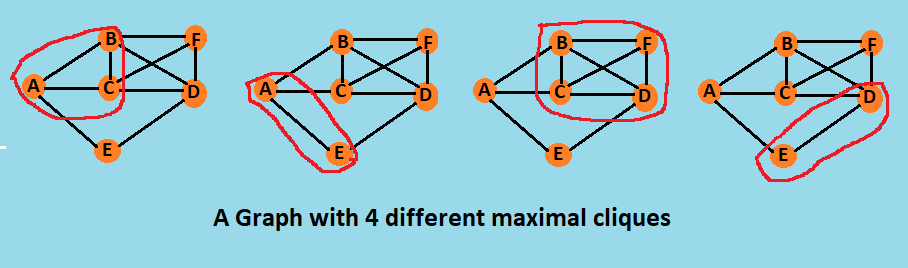×

Search anything:

# Greedy approach to find a single maximal clique in O(V^2) time complexity

#### Algorithms Greedy Algorithms cliqueGet this book -> Problems on Array: For Interviews and Competitive Programming

A clique is a subset of vertices of an undirected graph G such that every two distinct vertices in the clique are adjacent; that is, its induced subgraph is complete. Cliques are one of the basic concepts of graph theory and are used in many other mathematical problems and constructions on graphs.

A maximal clique is a clique that cannot be extended by including one more adjacent vertex, that is, a clique which does not exist exclusively within the vertex set of a larger clique. Maximal cliques can be very small. A graph may contain a non-maximal clique with many vertices and a separate clique of size 2 which is maximal. While a maximum (i.e., largest) clique is necessarily maximal, the converse does not hold.

There can be more than one single maximal clique in a non-complete graph (since complete graph is a maximal clique itself). To find a single maximal clique in a graph we use a straightforward greedy algorithm.

# Algorithm

In greedy approach of finding a single maximal clique we start with any Starting with an arbitrary clique (for instance, any single vertex or even the empty set), grow the current clique one vertex at a time by looping through the graph's remaining vertices.

For each vertex v that this loop examines, add v to the clique if it is adjacent to every vertex that is already in the clique, and discard v otherwise. The maximal clique can be different if we start with differnet vertex as a graph may have more than one maximal clique.

• Start from an arbitrary vertex
• Given a clique of size, repeat:
• Add a vertex randomly from the common neighbors of the existing clique
• If there is no common neighbors, stop and return the clique

The above algorithm runs in linear time in the size of the graph (Θ(n2) edges) because of:

• the ease of finding maximal cliques
• their potential small size

Following is an example graph with 6 vertices and 4 different maximal cliques. On selection of different arbitrary start vertex we may get different maximal clique as output of the algorithm.# Implementation

Code in Python3

``````from collections import defaultdict
import random

def find_single_clique(graph):
clique = []
vertices = list(graph.keys())
rand = random.randrange(0, len(vertices), 1)
clique.append(vertices[rand])
for v in vertices:
if v in clique:
continue
isNext = True
for u in clique:
if u in graph[v]:
continue
else:
isNext = False
break
if isNext:
clique.append(v)

return sorted(clique)

graph = dict()
graph['A'] = ['B', 'C', 'E']
graph['B'] = ['A', 'C', 'D', 'F']
graph['C'] = ['A', 'B', 'D', 'F']
graph['D'] = ['C', 'E', 'B', 'F']
graph['E'] = ['A', 'D']
graph['F'] = ['B', 'C', 'D']

clique = find_single_clique(graph)
print('A maximal clique in the graph is: ', clique)
``````

Output

``````A maximal clique in the graph is:  ['B', 'C', 'D', 'F']
``````

# Complexity

Time Complexity

• The greedy algorithm takes O(n2) (i.e. O(Edges)) time in the worst case.

Space Complexity

• The greedy algoorithm takes O(n2) auxiliary space in the worst case.
Using Bron Kerbosch algorithm to find maximal cliques in O(3^(N/3))
Algorithm to find cliques of a given size k 【O(n^k) time complexity】